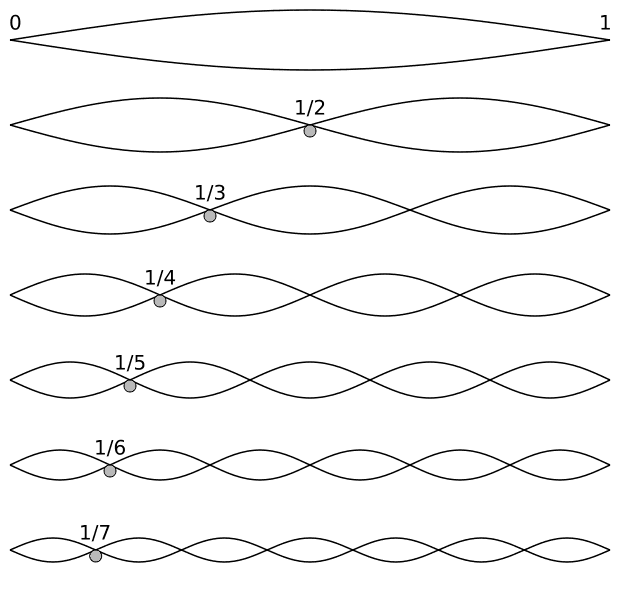# Quantized energy in infinite potential well

How does energy become quantized in an infinite potential well??

## Answers and Replies

alxm
Science Advisor
How do the harmonics of a finite string become quantized?

Honestly from a mathematical standpoint it comes about due to the boundary conditions of the Schrodinger equation for an infinite potential well.

How does energy become quantized in an infinite potential well??

The proper functions (eigenfunctions) of the Hamiltonian are sin(πnz) where z is the dimensionless length z=x/L. The proper values (eigenvalues) are proportional to (πn)2.

Any, I repeat, any wave inside the well can be decomposed in a sum of proper waves with some amplitudes. In general case the wave energy is not certain but dispersed. Only in the eigenstates the energy is certain.

How does energy become quantized in an infinite potential well??

As the frequencies of a string in a guitar $$E_n = h v_n = n h v$$That is through (periodic) boundary conditions.

For this aspect, Pythagoras was the first to study a problem of QM mechanics.

http://img523.imageshack.us/img523/5874/pitagoradagafuriotheorixk5.jpg [Broken]

Last edited by a moderator:
Honestly from a mathematical standpoint it comes about due to the boundary conditions of the Schrodinger equation for an infinite potential well.

But what if the solution we assume of Schrodinger equation be in exponential form??

But what if the solution we assume of Schrodinger equation be in exponential form??

it is completely equivalent.

But what if the solution we assume of Schrodinger equation be in exponential form??

In order to satisfy the boundary conditions the two complex exponentials have to have certain coefficients that make their sum to be sin(pi*n*x/L).